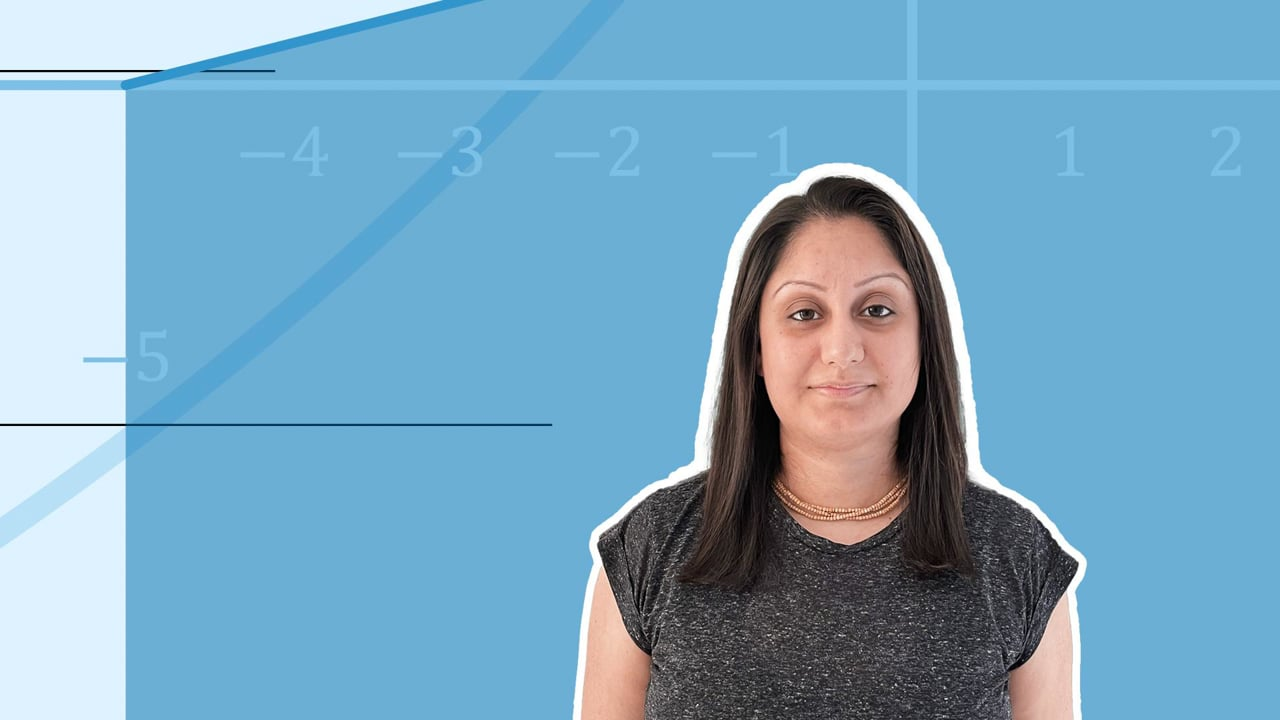Chapter overviewMaths

Exam board

AQA

Number

Algebra

Graphs

Ratio proportion and rates of change

Shapes and area

Angles and geometry

Trigonometry

Probability

Statistics

Maths

# Double and triple brackets - Higher0%

Summary

# Double and triple brackets

## In a nutshell

Double brackets consist of two binomial expressions multiplied together, e.g. $(x+1)(y-3)$, whereas triple brackets consist of three binomial expressions multiplied together, e.g. $(x-2)(x+6)(x+9)$. The principle behind multiplying out double brackets can be extended to multiplying out triple brackets, using the FOIL method, or a multiplication grid.

## Recap on multiplying double brackets

You can expand two pairs of brackets by multiplying each term in the first set of brackets by each term in he second set. This can be done by using a multiplication grid.

##### Example 1

Expand the brackets $(x+1)(2x-3)$

Fill in the multiplication grid

 $\times$​​ ​$\boldsymbol{x}$​​ ​$\bold{+1}$​​ ​$\boldsymbol{2x}$​​ ​$2x^2$​​ ​$+2x$​​ ​$\bold{-3}$​​ ​$-3x$​​ ​$-3$​​

\begin {aligned} (x+1)(2x-3) &= 2x^2 +2x-3x-3 \\&= \underline{2x^2-x-3}\end {aligned}

Note: when there are just two binomial expressions, you can also use the FOIL method, as discussed in the previous lesson (multiply the First terms, the Outer terms, Inside terms and the Last terms).

## Expanding Triple Brackets

To expand three brackets, first expand two of the brackets, then use the result to expand with the final pair. Use a multiplication grid to help.

##### Example 2

Expand $(x+1)(x+2)(x+3)$

Take the first two brackets, $(x+1)(x+2)$ and multiply out using a grid.
 ​$\times$​​ ​$\boldsymbol{x}$​​ ​$\bold{+1}$​​ ​$\boldsymbol{x}$​​ ​$x^2$​​ ​$+x$​​ ​$\bold{+2}$​​ ​$+2x$​​ ​$+2$​​
\begin {aligned} (x+1)(x+2) &= x^2 +x+ 2x+ 2 \\ &= x^2 + 3x +2 \end {aligned}
$(x+1)(x+2)(x+3) = (x^2 + 3x+2)(x+3)$